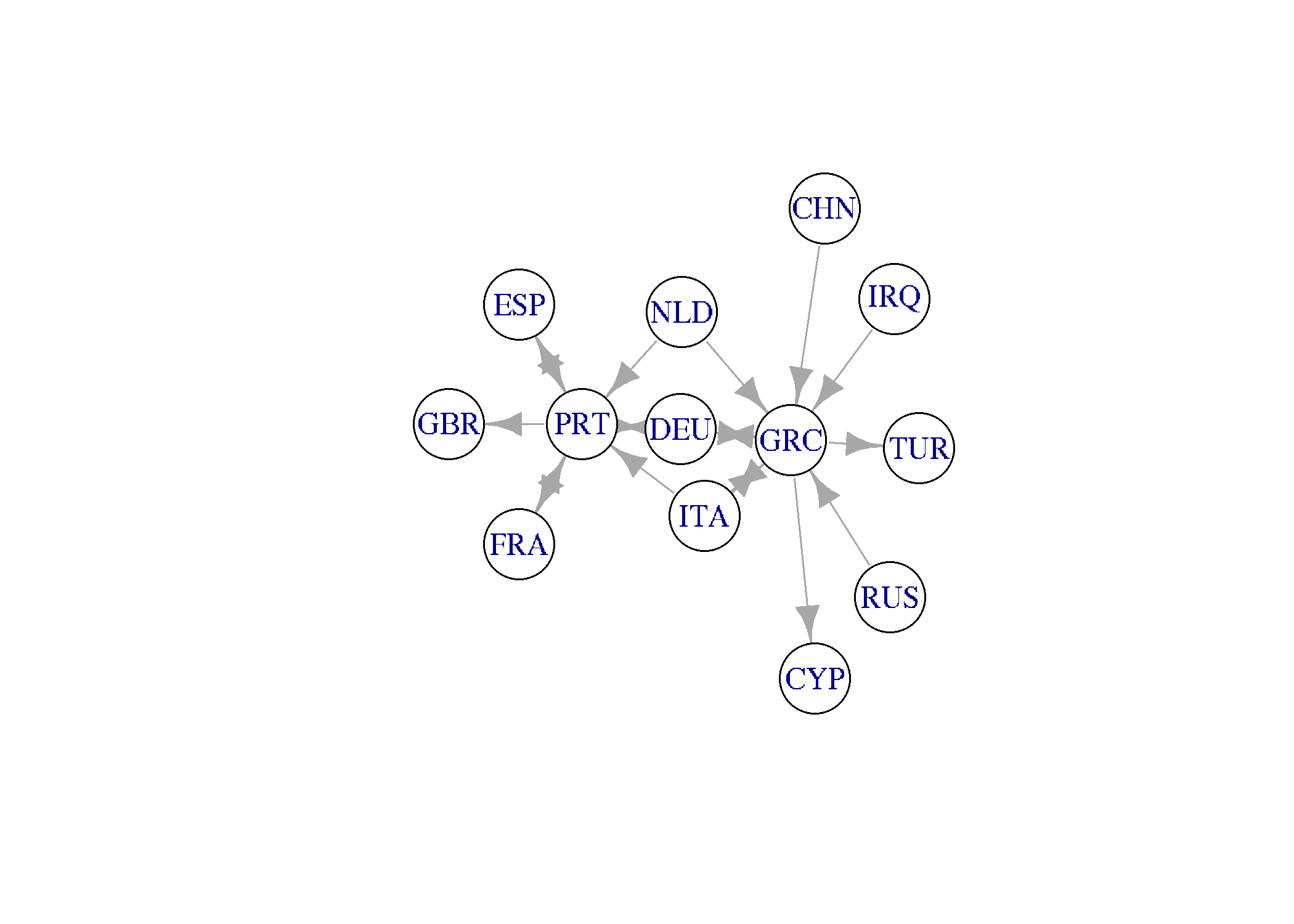Η ώρα είναι:

Comtrade is the UN database about international trade. It covers all countries, imports and exports, goods and services, all product categories (HS and SITC classifications), for more than 50 years.

The database is huge. It is not easy, from technical point of view, to download the whole content.

Comtrade has an excellent API, beyond its web interface. API, both with sdmx and R package, allows to download part of the database based on user’s queries.

This is exactly what we are going to do: Examine EU countries trade volumes in years 2013 and 2016. We will consider the total trade, not specific categories of products and services.

## Basic introduction

library(comtradr)
library(dplyr)
library(tidyr)
library(igraph)
library(ggplot2)
library(ggthemes)
library(DT)
library(haven)

comtradr is the basic library we use here: R’s interface to comtrade database. It provides functions to search and access the comtrade database.

Visit UN comtrade for more details

Here is a basic example:

ct_search(reporters = "Greece",
partners = c("Germany", "Italy", "Turkey"),
start_date = 2016,
end_date = 2016)

The resuted table contains many columns. Pay attention to trade_value_usd, it contains the value in USD of the tradde between an origin (reporter) and a destination (partner).

In any query term we can include multiple values, supplied as vectors. For example partners in the exampleabove.

However, too complex queries, or queries that return too many rows can cause problems and will not be processed by the server.

Since we do not have a subscription to get bulk data, we will split our queries in smaller ones.

But there is always a limit. You can check how remaining queries you have (hourly) and the reset time:

ct_get_reset_time()
 "2018-11-12 12:27:49 EET"
> ct_get_remaining_hourly_queries()
 94

## Compare Greece and Portugal

trade_13 <- ct_search(reporters = c("Greece", "Portugal"),
partners = "All",
type = c("goods", "services"),
start_date = 2013,
end_date = 2013)

trade_16 <- ct_search(reporters = c("Greece", "Portugal"),
partners = "All",
type = c("goods", "services"),
start_date = 2016,
end_date = 2016) 
trade_eu <- read_rds("../data/trade_eu.rds")
filter(year == 2013 & reporter %in% c("Greece", "Portugal"))
filter(year == 2016 & reporter %in% c("Greece", "Portugal"))

Exports of Greece in 2013:

trade_13 %>%
filter(reporter == 'Greece' & trade_flow == 'Export') %>%
datatable()

Now we can examine the total values for the two countries:

trade_grc_prt <- bind_rows(trade_13, trade_16)
filter(partner == 'World') %>%
datatable()

Or we can tranform the values to bilions USD, also we can omit some columns:

trade_grc_prt <- bind_rows(trade_13, trade_16)
filter(partner == 'World') %>%
datatable(trade_grc_prt)

## Plotting

A basic plot for Greece:

trade_grc_prt %>%
filter(reporter == 'Greece') %>%
ggplot() +
geom_col(aes(x = factor(year), y = trade_value,
fill = trade_flow), position = "stack")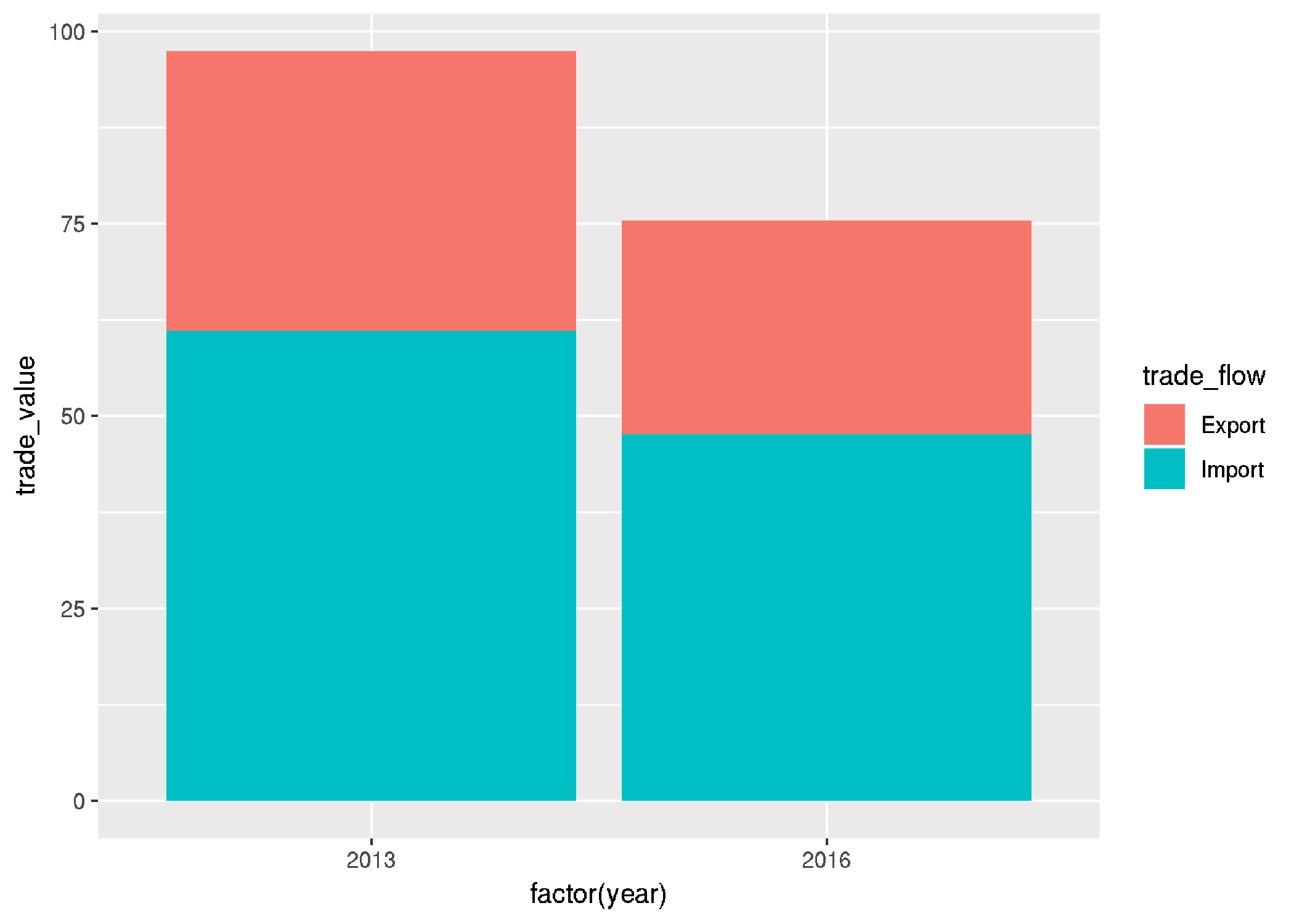Or side by side:

trade_grc_prt %>%
filter(reporter == 'Greece') %>%
ggplot() +
geom_col(aes(x = factor(year), y = trade_value,
fill = trade_flow), position = "dodge2")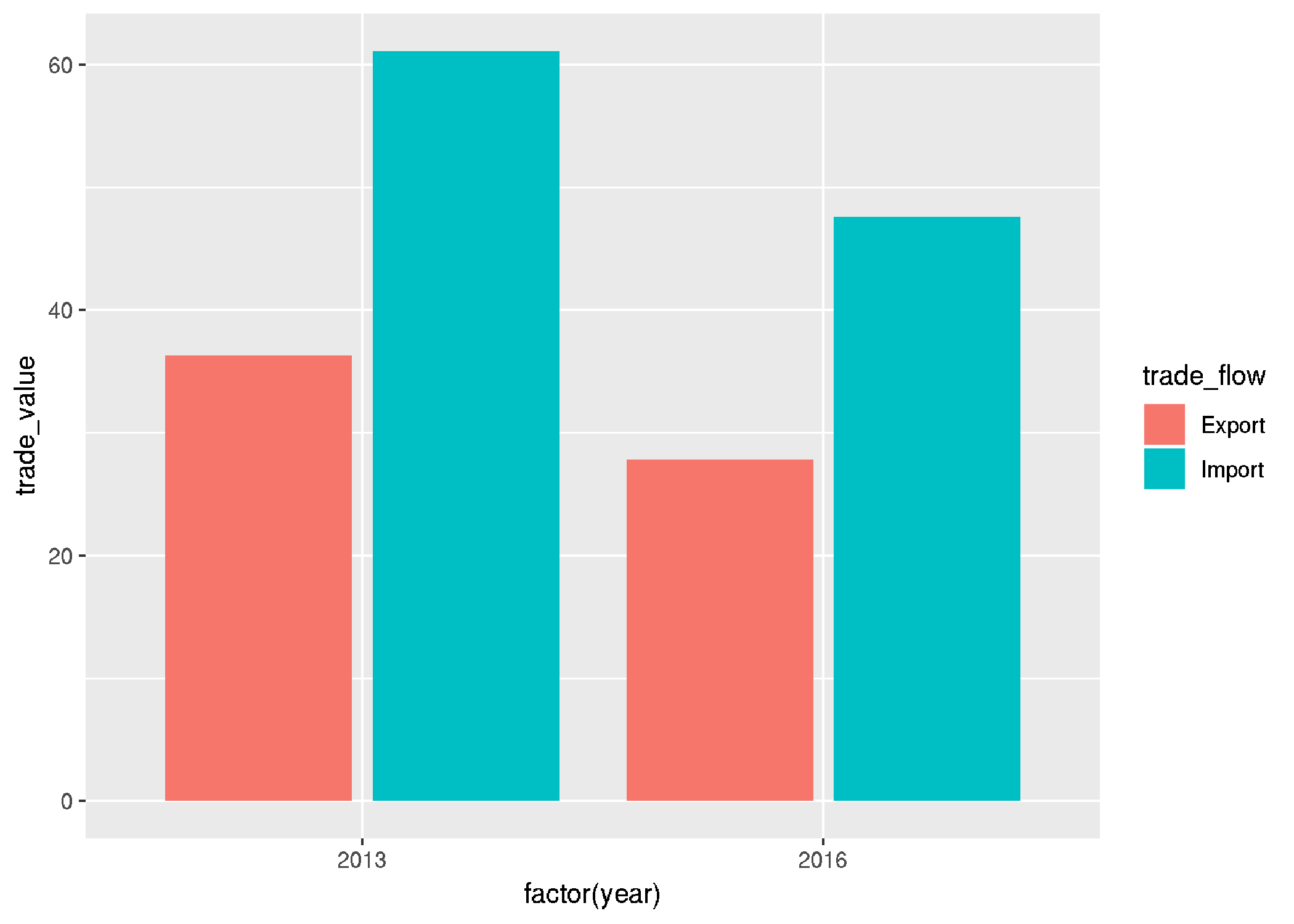Here is Portugal:

trade_grc_prt %>%
filter(reporter == 'Portugal') %>%
ggplot() +
geom_col(aes(x = factor(year), y = trade_value,
fill = trade_flow), position = "dodge2") +
labs(x = "Year", y = "trade value (b. USA)") +
theme_economist() +
theme(legend.title = element_blank()) +
theme(text = element_text(size = 14))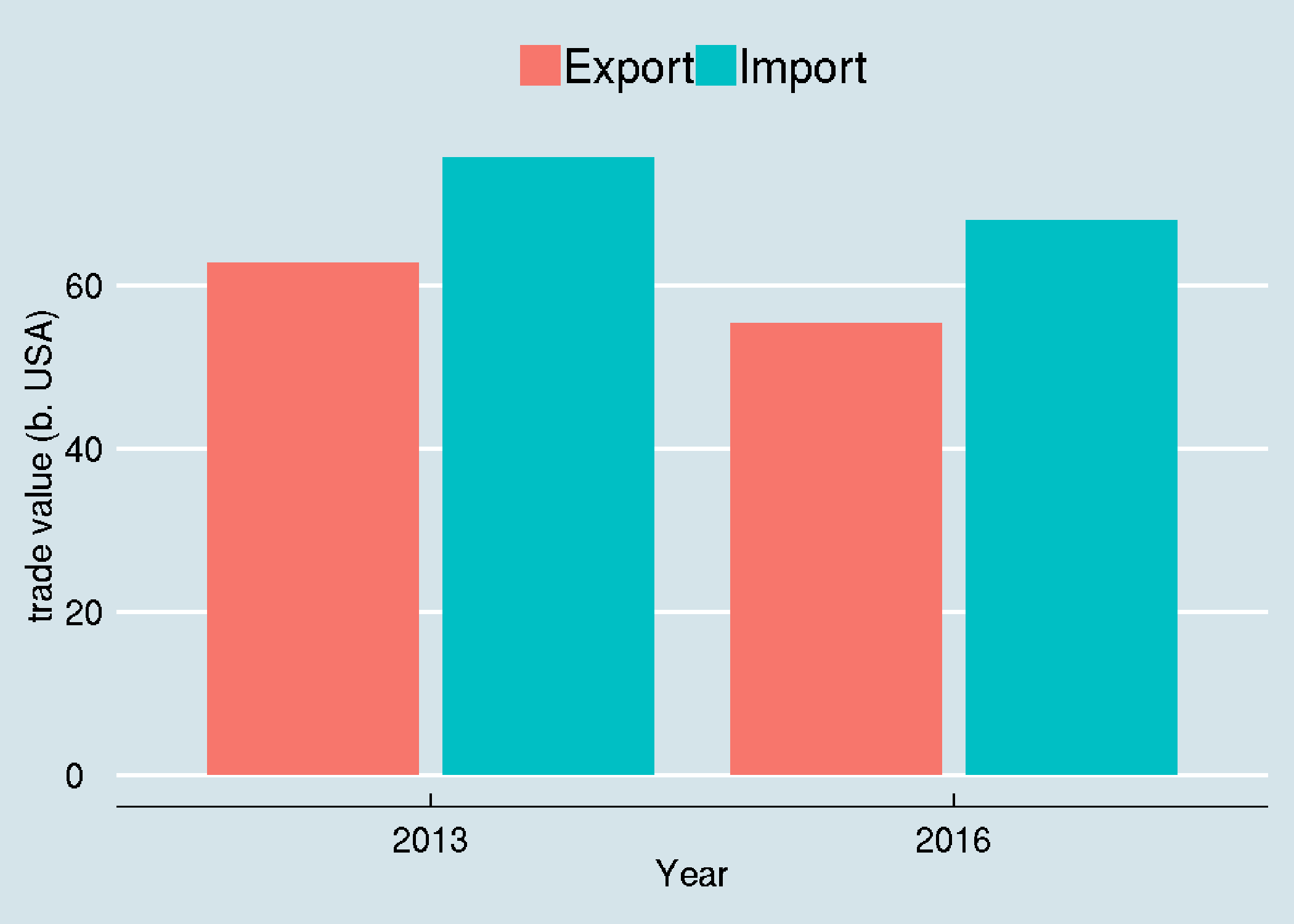Compare exports of two countries:

trade_grc_prt %>%
ggplot() +
geom_col(aes(x = factor(year), y = trade_value,
fill = reporter), position = "dodge2") +
labs(x = "Year", y = "trade value (b. USA)") +
theme_economist() +
theme(legend.title = element_blank()) +
theme(text = element_text(size = 14))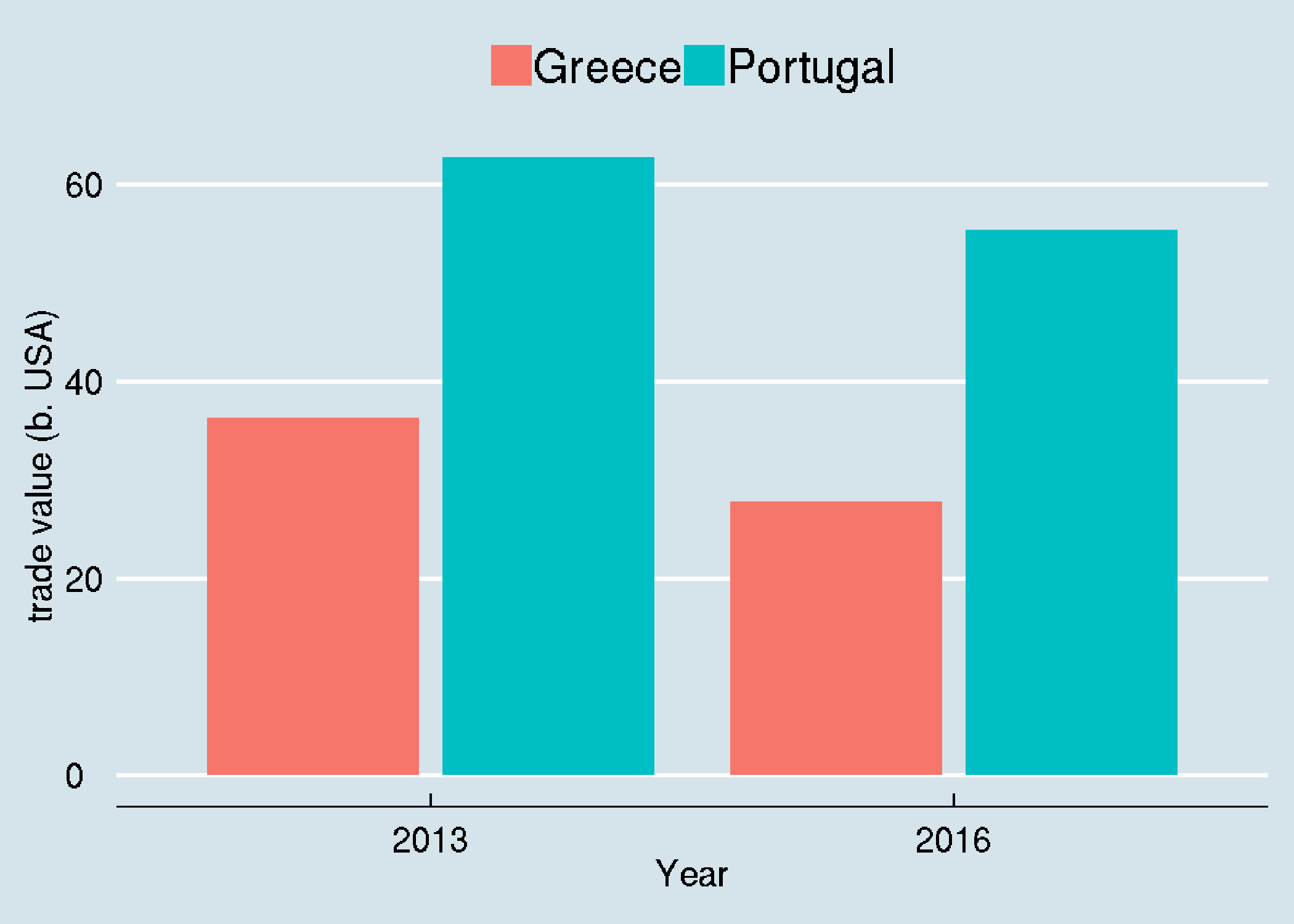Compare Exports and Imports in two countries simoultaneoudly

trade_grc_prt %>%
ggplot() +
geom_col(aes(x = factor(year), y = trade_value,
fill = reporter), position = "dodge2") +
labs(x = "Year", y = "trade value (b. USA)") +
theme_economist() +
theme(legend.title = element_blank()) +
theme(text = element_text(size = 14))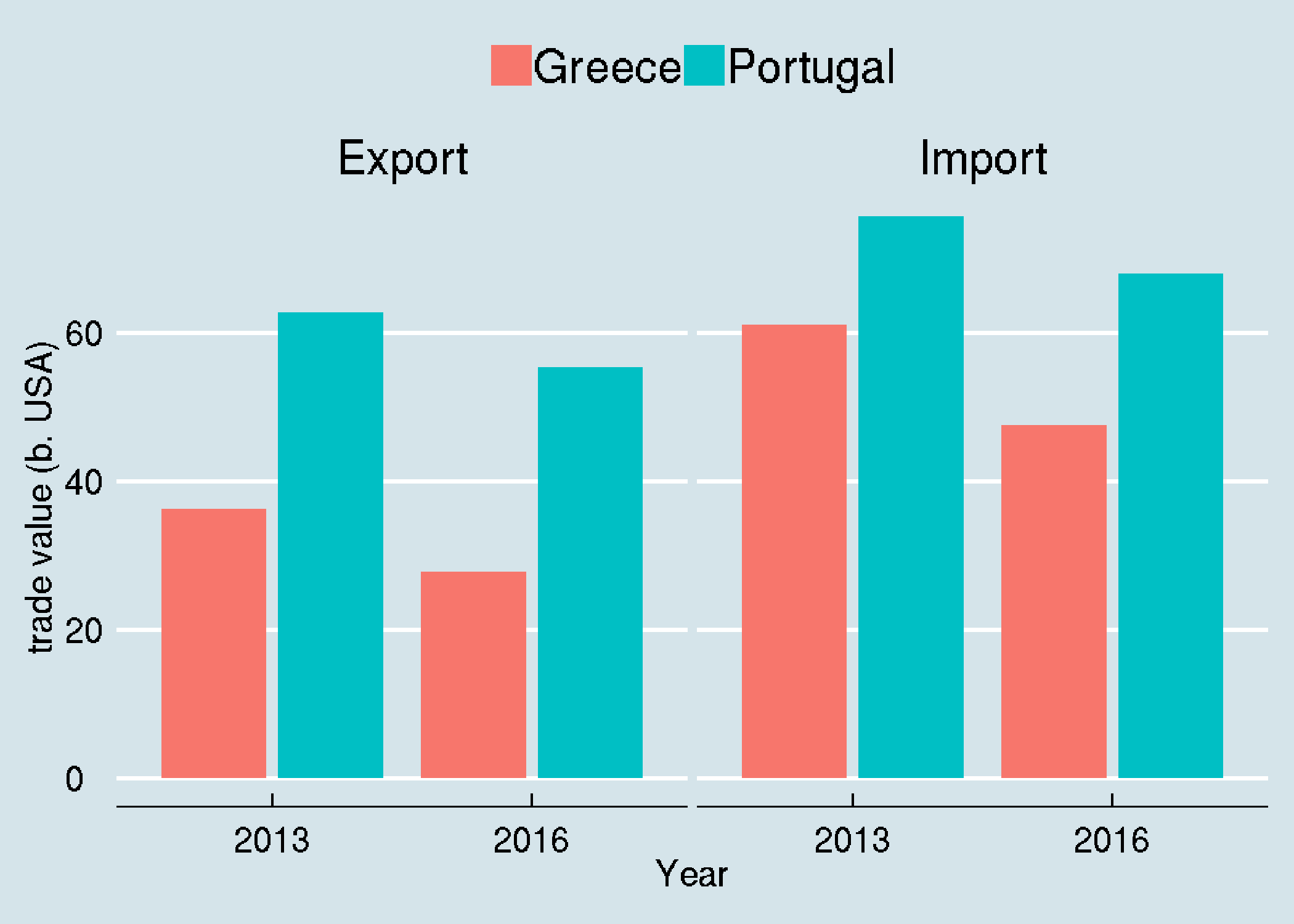trade_grc_prt %>%
ggplot() +
geom_col(aes(x = factor(year), y = trade_value,
fill = trade_flow), position = "dodge2") +
labs(x = "Year", y = "trade value (b. USA)") +
facet_wrap(~reporter) +
theme_wsj() +
theme(legend.title = element_blank()) +
theme(text = element_text(size = 14))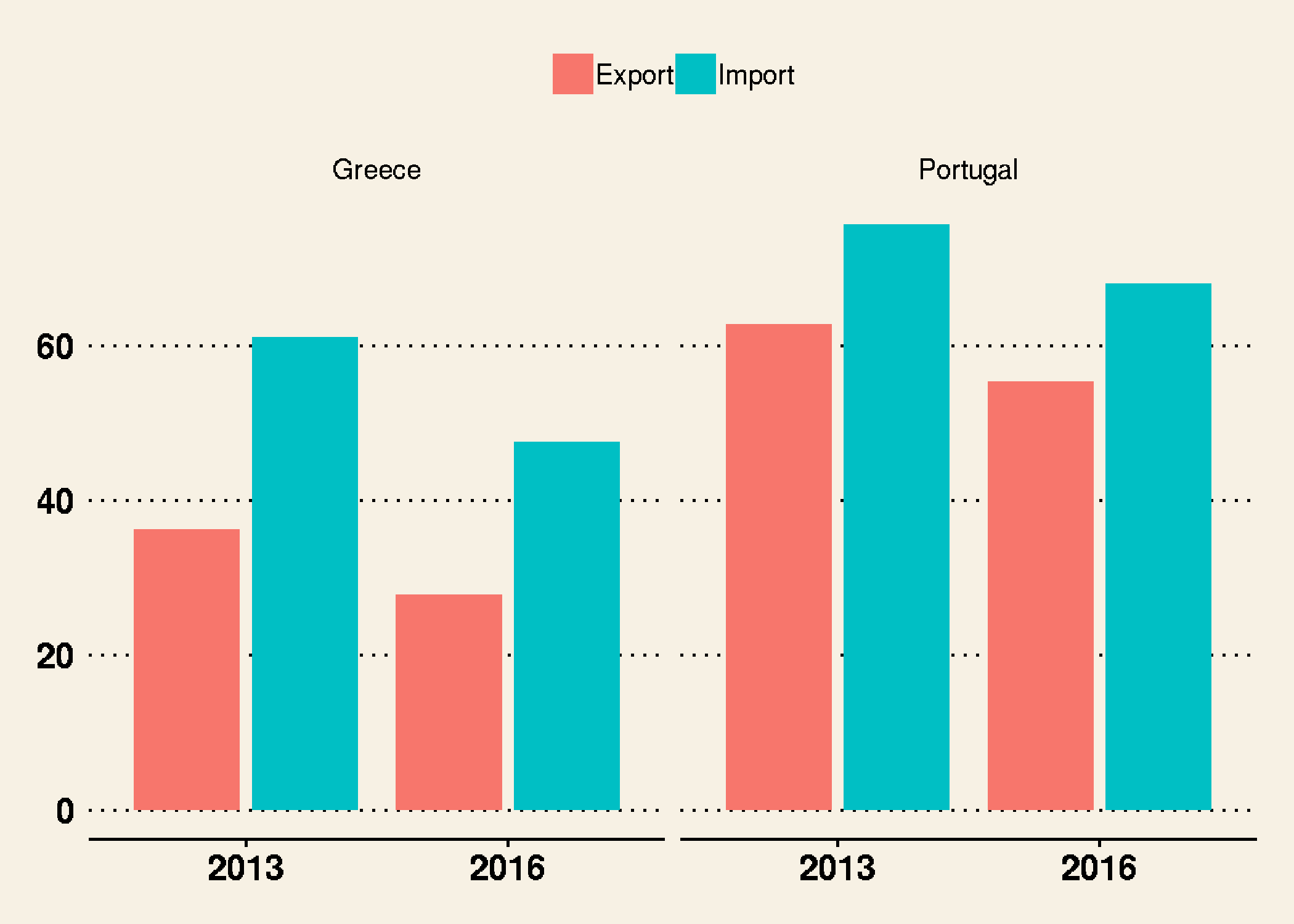trade_grc_prt %>%
mutate( Balance = Export - Import) %>%
ggplot() +
geom_col(aes(x = reporter, y = Balance,
fill = factor(year)), position = "dodge2") +
labs(x = NULL, y = "Value (b. USA)") +
coord_flip() +
theme_economist() +
theme(legend.title = element_blank()) +
theme(text = element_text(size = 14))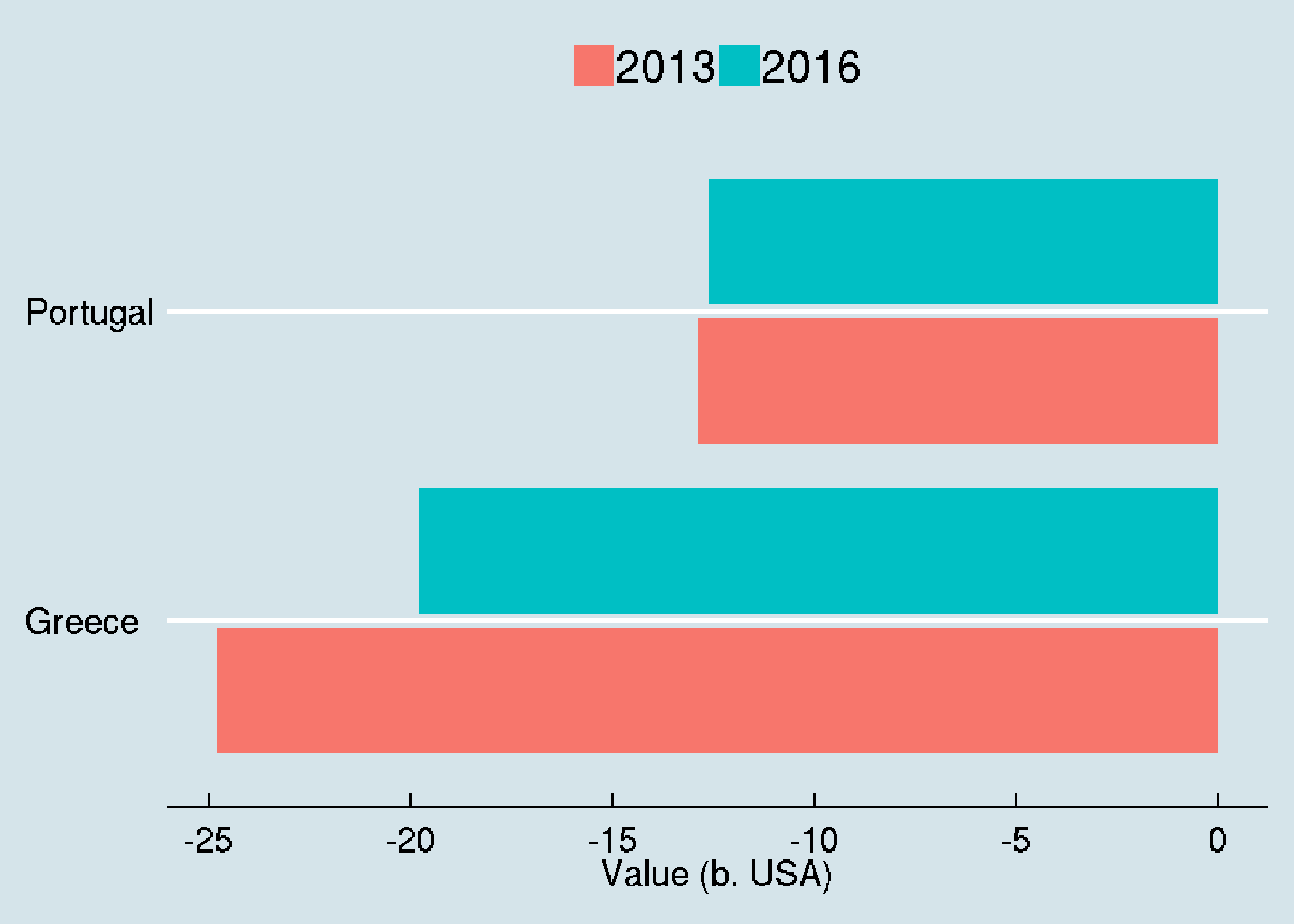As countries trade between each other they form a network of partnerns. We will use the igraph to demonsrate these interactions. We will also focus to main partners, thus we consider only cases where the trade share is more than 5%.

Here is the list of countries that Greece exported during 2016:

trade_graph <- trade_eu %>%
filter(partner != 'World' &  year == 2016
& reporter_iso == 'GRC' &
select(reporter_iso, partner_iso) %>%
distinct() %>%
graph.data.frame(directed = F)
plot(trade_graph, vertex.size= 40, vertex.color = "white")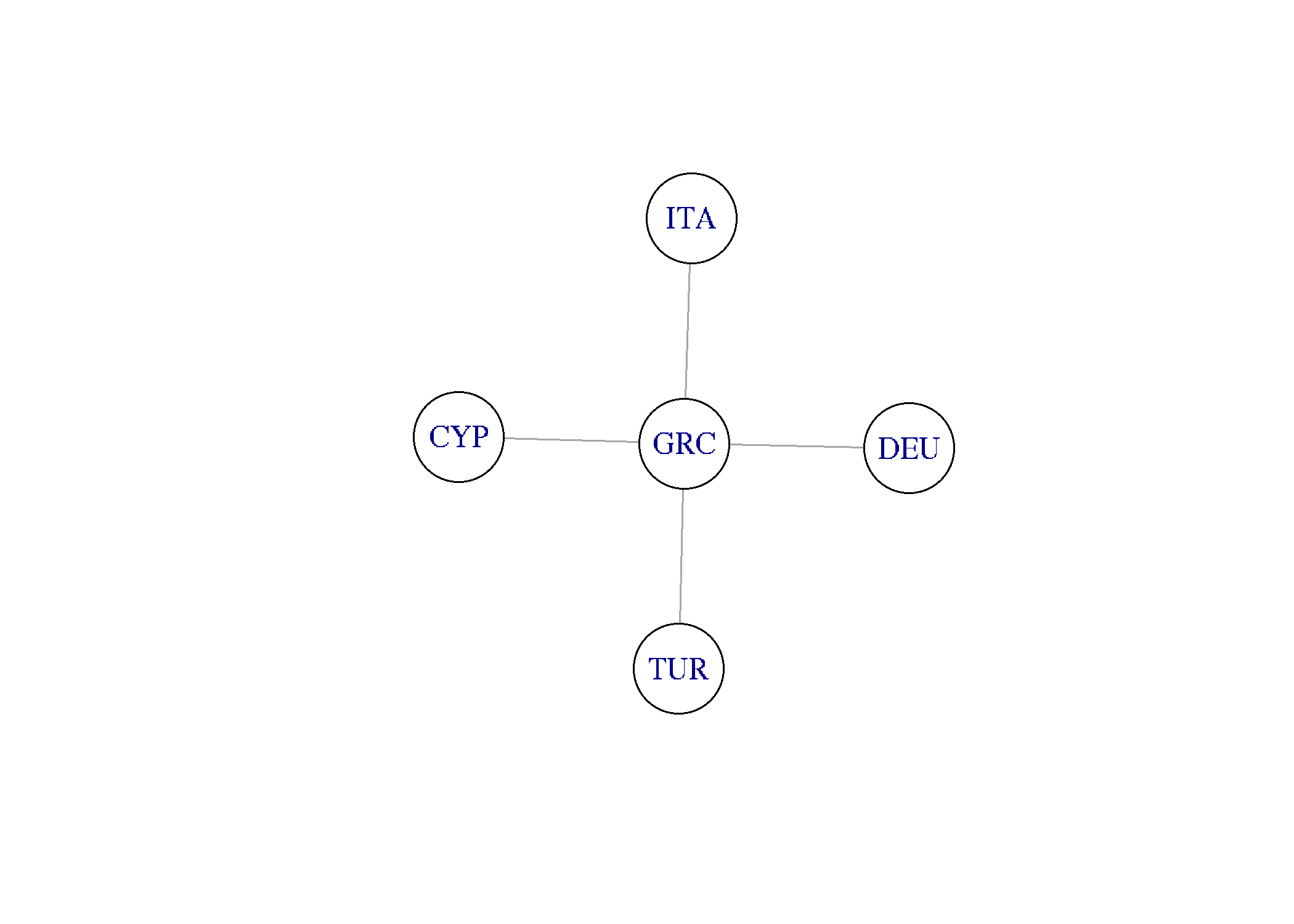And here is Portugal:

trade_graph <- trade_eu %>%
filter(partner != 'World' &  year == 2016
& reporter_iso == 'PRT' &
select(reporter_iso, partner_iso) %>%
graph.data.frame(directed = F)
plot(trade_graph, vertex.size= 40, vertex.color = "white")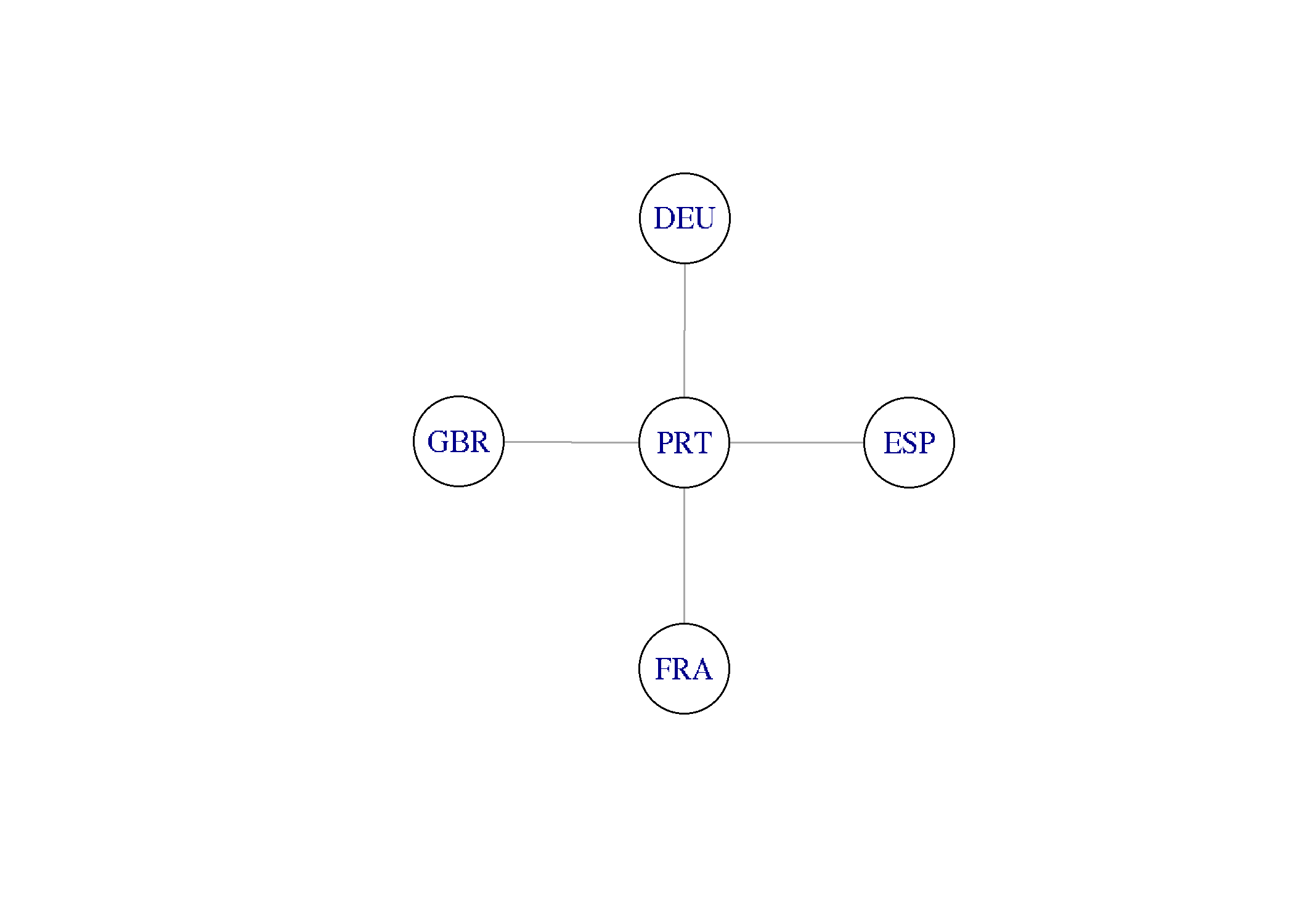As we can the two countries have different export partners. We can combine easily:

trade_graph <- trade_eu %>%
filter(partner != 'World' &  year == 2016
& reporter_iso %in% c('GRC', 'PRT') &
select(reporter_iso, partner_iso) %>%
graph.data.frame(directed = F)
plot(trade_graph, vertex.size= 40, vertex.color = "white")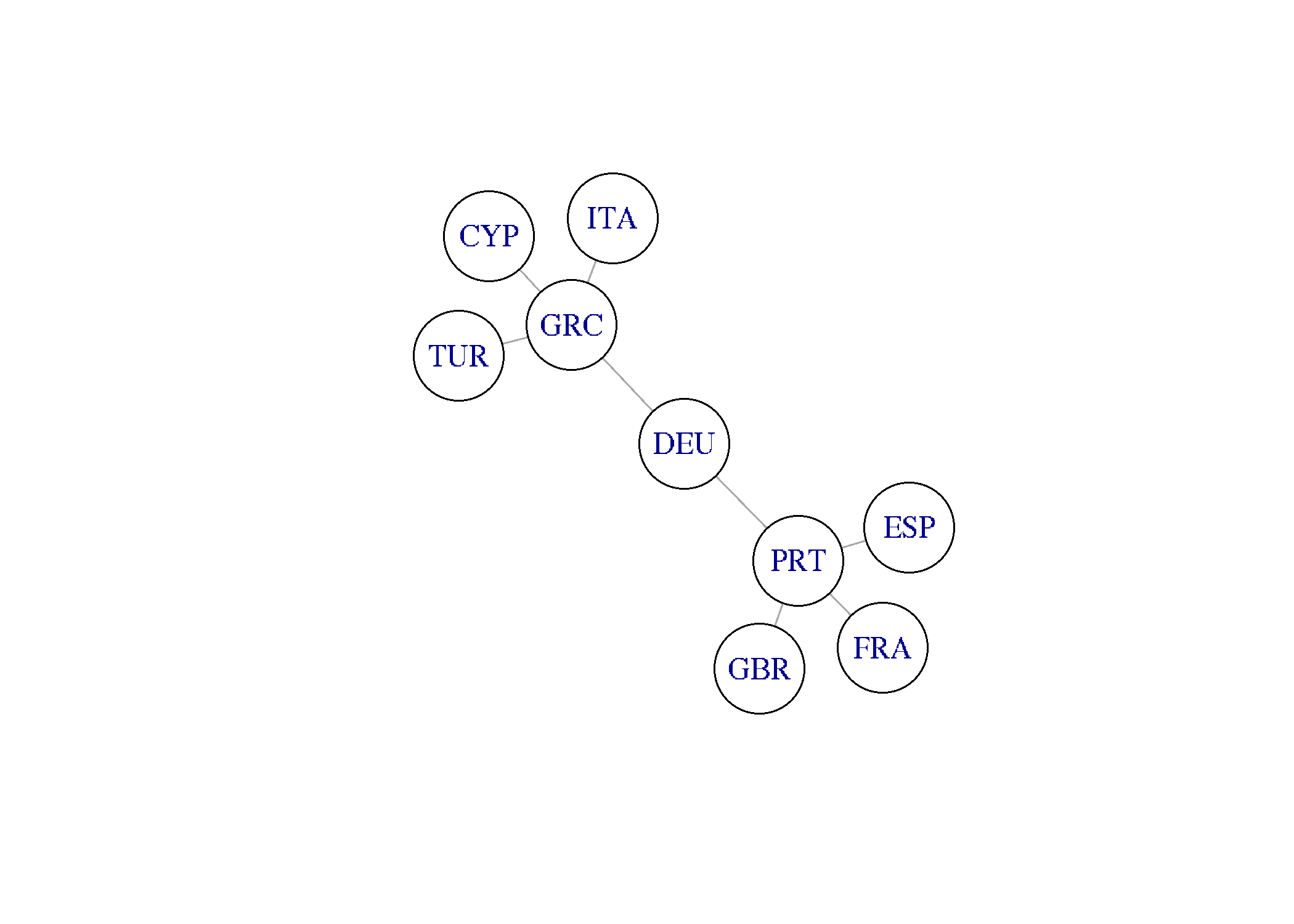Now,we can easily see Germany is important export destination for both Greece and Portugal.

We can produced an analogous graph for imports:

trade_graph <- trade_eu %>%
filter(partner != 'World' &  year == 2016
& reporter_iso %in% c('GRC', 'PRT') &
select(reporter_iso, partner_iso) %>%
graph.data.frame(directed = F)
plot(trade_graph, vertex.size= 40, vertex.color = "white")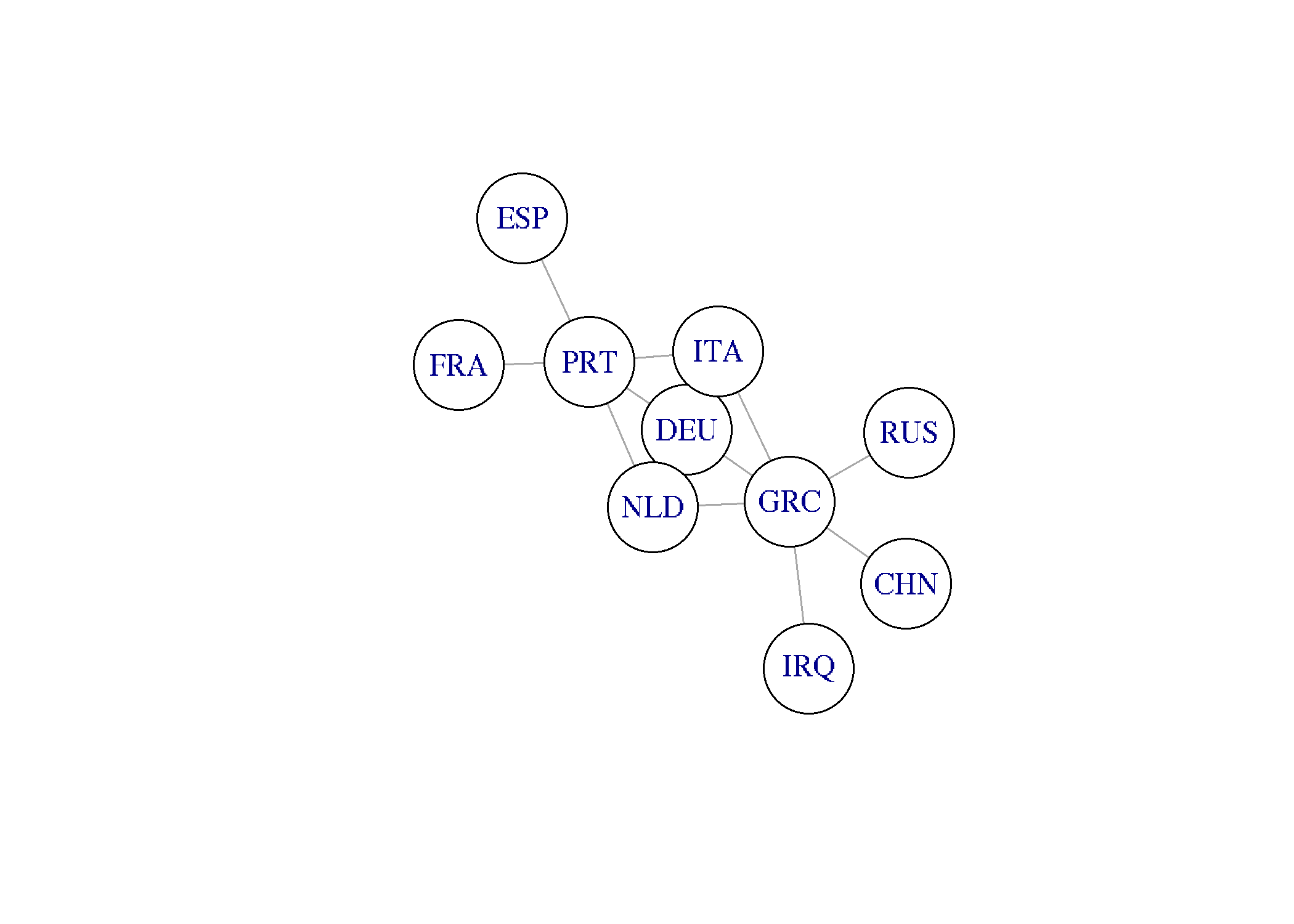And finally we can combine all in one directed network graph:

trade_ex <- trade_eu %>%
filter(partner != 'World' &  year == 2016
& reporter_iso %in% c('GRC', 'PRT') &
rename(origin_iso = reporter_iso, destination_iso = partner_iso) %>%
select(origin_iso, destination_iso)

filter(partner != 'World' &  year == 2016
& reporter_iso %in% c('GRC', 'PRT') &
rename(origin_iso = partner_iso, destination_iso = reporter_iso) %>%
select(origin_iso, destination_iso)
plot(graph_ex_im, vertex.size= 30, vertex.color = "white")